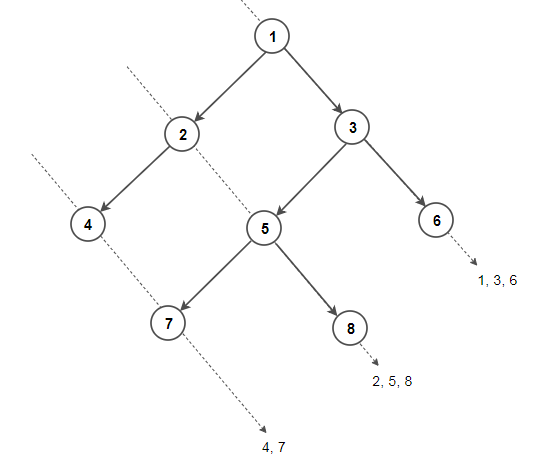# Print Diagonal Traversal of Binary Tree

Given a binary tree, print all nodes for each diagonal having negative slope (\). Assume that the left and right child of a node makes 45 degree angle with the parent.

For example, consider below binary tree having three diagonals (marked in red).
The diagonals traversal is

1 3 6
2 5 8
4 7This problem can be easily solved with the help of Hashing. The idea is to create an empty map where each key in the map represents a diagonal in the binary tree and its value maintains all nodes present in the diagonal. Then we do a pre-order traversal of the tree and update the map. For each node, we recurse for its left subtree by increasing diagonal by 1 and recurse for right subtree with same diagonal.

## C++

The time complexity of above solution is O(nlog(n)) and auxiliary space used by the program is O(n).

##### Iterative version –

We can also use Queue to solve this problem. The idea is similar to level order traversal but instead of storing nodes of a level, we enqueue nodes in a diagonal.

## C++

The time complexity of above solution is O(n) and auxiliary space used by the program is O(n).

Exercise: Modify the solution to print diagonal elements for diagonals having positive slope (/).(1 votes, average: 5.00 out of 5)Loading...

Please use our online compiler to post code in comments. To contribute, get in touch with us.
Like us? Please spread the word and help us grow. Happy coding 🙂Subscribe
Notify ofGuest
EyalMendel

Another iterative solution will be using a queue to save ONLY the left child of each visited node (if there is one).
Starting from the root, on each visited node do the following:
1. Do the work (print the data for this manner)
2. If left child exists, push to queue
3. If right child exists, current = current->right
else, current = q.pop()
4. repeat while queue is not emptyGuest
Dibyendu

Hi,

I think the time complexity of the recursive version is O(nlogn) as map has O(logn) complexity for each insertion/deletion/lookup. Please let me know if I’m wrong.

And also keep adding more algorithms. I find your code easy to understand, concise and up-to-date with c++14. Thanks!